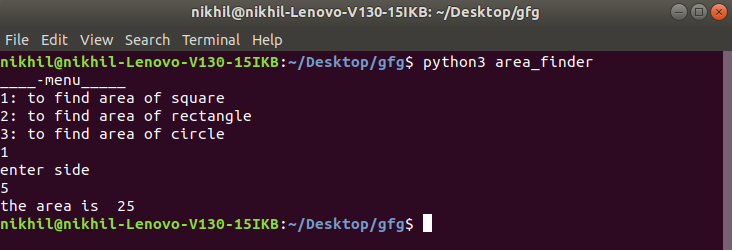Open In App

# Usage of __main__.py in Python

Many of us have worked with creating their own custom module in Python and is well familiar with the candidate ‘__init__.py’. If you do not know then let’s get a brief and short description of ‘__init__.py’ before diving deep into the concerned topic. Actually, when we try to import a module to Python by default the __init__.py is accessed by Python to look for the necessary imports. Now there are two scenarios.

• Python files which are well organized to be imported as modules
• Python files (single file structure) which can be run directly by command line

In the first scenario when we try to import it as a module __init__.py comes into play. For the second scenario we use some hackish syntax like

## Python3

 `def` `main ():``    ``pass` `if` `__name__ ``=``=` `'__main__'``:``    ``main()`

This syntax can be related to starting of main in other languages like JAVA and C++. But this syntax will not work ideally with the third scenario. Then what to do if we want to run such files directly from command line ? This is where __main__.py comes to rescue. Let’s take an example, we will find the area of a rectangle, square, and circle. For the sake of understanding, let’s divide the three operations under three separate .py files and store it in a module named src. The file tree will look like this

```+---src
|   |   circle.py
|   |   rectangle.py
|   |   square.py
|   |   __init__.py
|   |   ```

Now src can be imported as a module from any other Python program if added to path. But what if we want to run it from command line. For this we store the src folder under a folder named say area_finder and add a file named __main__.py under it . The tree would look like

```area_finder
|   __main__.py
|
+---src
|   |   circle.py
|   |   rectangle.py
|   |   square.py
|   |   __init__.py
|   |   ```

content of __main__.py

## Python3

 `print``("____``-``menu_____")``print``("``1``: to find area of square \n\``2``: to find area of rectangle\n\``3``: to find area of circle")` `ch ``=` `int``(``input``())` `if` `ch ``=``=` `1``:``    ``from` `src.square ``import` `square``    ``print``("enter side")``    ``s ``=` `int``(``input``())``    ``print` `("the area ``is` `", square(s))` `if` `ch ``=``=` `2``:``    ``from` `src.rectangle ``import` `rectangle``    ``print``("enter length ``and` `breadth")``    ``l ``=` `int``(``input``())``    ``b ``=` `int``(``input``())``    ``print``("the area ``is` `", rectangle(l, b))` `if` `ch ``=``=` `3``:``    ``from` `src.circle ``import` `circle``    ``print``("enter radius")``    ``r ``=` `int``(``input``())``    ``print``("the area ``is` `", circle(r))`

Now when we run them from terminal/command prompt like this

`python area_finder`

The output will be –So what happens when we execute the command. Python looks for a file named __main__.py to start its execution automatically. If it doesn’t find it will throw an error else it will execute main.py and from the code, you can well understand that it will import the modules from src to find the area. So now we have learned how __main__.py works. Now, let’s take a look at its biggest advantages:

• It removes the ambiguity among end-user about the entry point of the program as Python does it automatically
• It helps in clean execution of the code